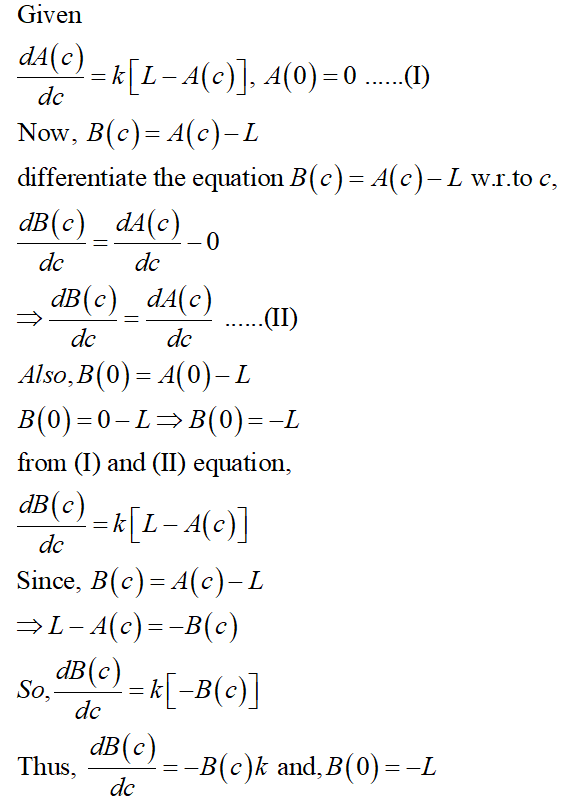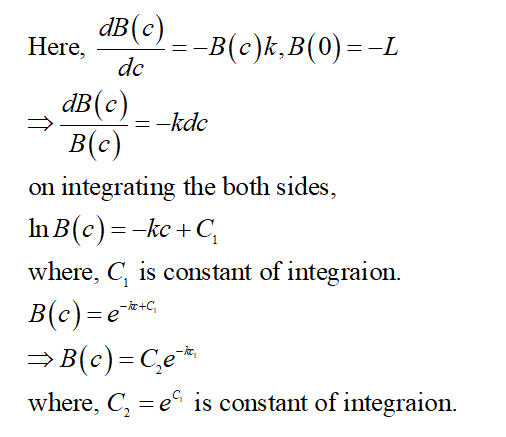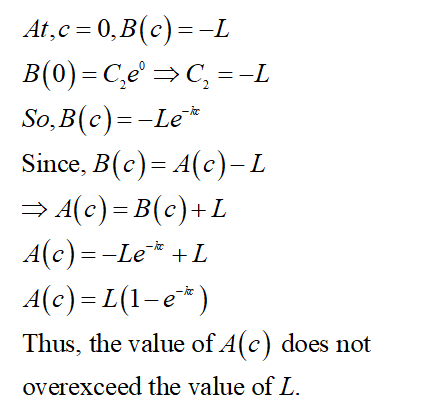# Active oxygen and free radicals are believed to be exacerbating factors in causing cell injury and aging in living tissue . Researchers are therefore interested in understanding the protective role of natural antioxidants. In the study of one such antioxidant (Hsian-tsao leaf gum), the antioxidation activity of the substance has been found to depend on concentration in the following way: dA(c)\dc=k[L−A(c)],   A(0)=0.dA(c)dc=k[L−A(c)],   A(0)=0.In this equation, the dependent variable AA is a quantitative measure of antioxidant activity at concentration cc. The constant LL represents a limiting or equilibrium value of this activity, and kk a positive rate constant. Let B(c)=A(c)−L and reformulate the given initial value problem in terms of this new dependent variable, B.dB(c)/dc=  B(0)=Solve the new initial value problem for B(c)and then determine the quantity A(c).A(c)= Does the activity A(c) ever exceed the value L?  ? yes no Determine the concentration at which 95% of the limiting antioxidation activity is achieved. (Your answer is a function of the rate constant kk.)c=

Question
616 views

Active oxygen and free radicals are believed to be exacerbating factors in causing cell injury and aging in living tissue . Researchers are therefore interested in understanding the protective role of natural antioxidants. In the study of one such antioxidant (Hsian-tsao leaf gum), the antioxidation activity of the substance has been found to depend on concentration in the following way:

dA(c)\dc=k[L−A(c)],   A(0)=0.dA(c)dc=k[L−A(c)],   A(0)=0.

In this equation, the dependent variable AA is a quantitative measure of antioxidant activity at concentration cc. The constant LL represents a limiting or equilibrium value of this activity, and kk a positive rate constant.

1. Let B(c)=A(c)−L and reformulate the given initial value problem in terms of this new dependent variable, B.

dB(c)/dc=

B(0)=
2. Solve the new initial value problem for B(c)and then determine the quantity A(c).

A(c)=
Does the activity A(c) ever exceed the value L?  ? yes no

3. Determine the concentration at which 95% of the limiting antioxidation activity is achieved. (Your answer is a function of the rate constant kk.)

c=
check_circle

Step 1

Consider the given questions,

(1)Step 2

(2)

Now, solve the new initial value problem for B(c) and then find the quantity A(c).Step 3

Further simplify the above equation,...

### Want to see the full answer?

See Solution

#### Want to see this answer and more?

Solutions are written by subject experts who are available 24/7. Questions are typically answered within 1 hour.*

See Solution
*Response times may vary by subject and question.
Tagged in

### Math